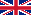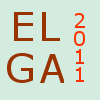ELGA 2011Cursos para Estudiantes

### Cursos para Estudiantes

Durante la primera semana (1-5 de agosto) se van a dictar 4 cursos para estudiantes en Buenos Aires. Horarios.

Cada curso tendrĂˇ 5 clases de entre 60 y 90 minutos que, en algunos casos, incluirĂˇn ejercicios a ser resueltos por los alumnos. Todas las clases serĂˇn en inglĂ©s.

Los primeros dos cursos tratan temas bĂˇsicos de GeometrĂ­a Algebraica y tienen aplicaciones a la FĂ­sica, Sistemas DinĂˇmicos y CĂˇlculos efectivos. El tercer curso es sobre un tema emergente, con aplicaciones a la Conjetura Espejo, degeneraciĂłn de variedades algebraicas, EstadĂ­stica Algebraica, etc. El cuarto curso es sobre el tema bien establecido de la aplicaciĂłn de la teorĂ­a de curvas algebraicas sobre cuerpos finitos.

### Curso 1

#### Intersection Theory

Lecturer: Joe Harris (Harvard)

1. The basics of intersection theory and its applications: cycles, rational equivalence, intersection products, pushforwards and pullbacks; applications to the geometry of Veronese varieties, Segre varieties and plane cubics.

2. Chern classes: motivation, definition and key properties; applications to linear spaces on hypersurfaces and singular elements of linear series.

3. Parameter spaces: the choice of parameter space in solving an enumerative problem; application to the 5-conic problem.

4. Projective bundles: Chow ring of a projective bundle and the classes of its tangent bundle; applications to the 8-conic problem and secant varieties to rational curves.

5. Excess intersection: examples in low dimension, proofs in special cases and a proof in general; applications to the Chow ring of a blow-up and the double point formula.

### Curso 2

#### Introduction to Deformation Theory

Lecturer: Robin Hartshorne (UC Berkeley)

Abstract: This will be an introductory course, assuming only a basic knowledge of algebraic geometry in the language of schemes and cohomology. Topics will include the notion of a deformation as a flat family; first-order deformations of subschemes, abstract varieties, line bundles, vector bundles; higher-order deformations and the formal notion of pro-representable functors; the problem of global moduli and various extensions of the concept of scheme--algebraic spaces, stacks to accommodate the spaces that arise as moduli. Many examples will be given to illustrate these concepts.

Exercises

### Curso 3

#### Introduction to Tropical Algebraic Geometry

Lecturer: Diane Maclagan (Warwick)

Abstract: This course will provide an elementary introduction to the emerging field of tropical algebraic geometry, where a variety is replaced by a combinatorial shadow, known as the tropical variety. The lectures will define these objects, and explore what is preserved under this "tropicalization" procedure. No prior knowledge of algebraic geometry will be assumed. A preliminary outline of the course is as Ă‚Â follows:

• Lecture 1: Introduction to tropical mathematics.
• Lecture 2: Alternative descriptions of tropical varieties.
• Lecture 3: The combinatorial structure of tropical varieties.
• Lecture 4: Tropical geometry and toric geometry.
• Lecture 5: Applications to birational geometry or implicitization.

Note: Professor Maclagan is preparing a book on Tropical Geometry, jointly with Bernd Sturmfels.

### Curso 4

#### Algebraic Geometric Codes

Lecturer: Peter Beelen (Copenhaguen)

Abstract: In this course we will introduce algebraic geometry codes, discuss their construction and their decoding. Since the introduction of these codes in the 1980's many developments took place. In the first place the underlying algebraic objects, namely algebraic curves and varieties with many points have been understood in a better and more explicit way. In the second place faster decoding algorithms have become available. In the course we will discuss some of these explicit constructions, among others asymptotically optimal towers of function fields. Also we will consider several decoding algorithms, among others the majority voting algorithm and the Guruswami-Sudan list-decoding algorithm. When time allows we will also mention some more recent applications to cryptography.

Última actualización : agosto 2011